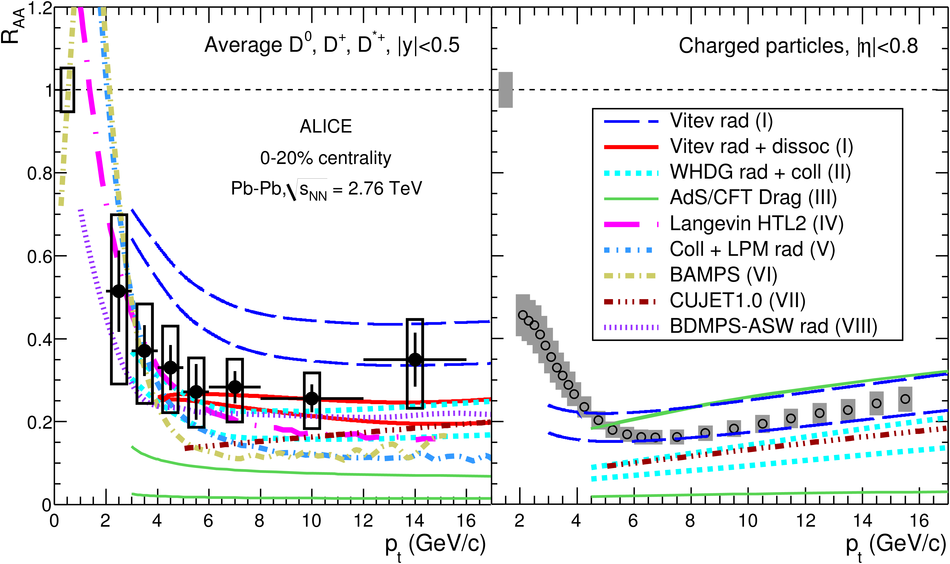# Suppression of high transverse momentum D mesons in central Pb-Pb collisions at $\sqrt{s_{\rm NN}}=2.76$ TeV

The production of the prompt charm mesons $D^0$, $D^+$, $D^{*+}$, and their antiparticles, was measured with the ALICE detector in Pb-Pb collisions at the LHC, at a centre-of-mass energy $\sqrt{s_{NN}}=2.76$ TeV per nucleon-nucleon collision. The $p_{\rm T}$-differential production yields in the range $2<~p_{\rm T}<~16$ GeV/c at central rapidity, $|y|<~0.5$, were used to calculate the nuclear modification factor $R_{AA}$ with respect to a proton-proton reference obtained from the cross section measured at $\sqrt{s}=7$ TeV and scaled to $\sqrt{s}=2.76$ TeV. For the three meson species, $R_{AA}$ shows a suppression by a factor 3-4, for transverse momenta larger than 5 GeV/c in the 20% most central collisions. The suppression is reduced for peripheral collisions.

Figures

## Figure 1

 Left: track impact parameter resolution in the transverse plane asa function of $\pt$ in Pb-Pb collisions. For $\pt< 2~\gev/c$, pion identification by the TPC or TOF detectors is required; the results for data and simulation are shown The simulation includes the effect of the residual geometrical misalignment ofthe sensor modules of the Inner Tracking System (see text for details). Right: difference between the measured time-of-flight and that expected under the kaon hypothesis as a function of track momentum for the 20% most central Pb-Pb collisions.## Figure 2

 Invariant mass distributions for $\Dzero$ (upper panels), $\Dplus$ (central panels), and $\Dstar$ (lower panels) candidates and theircharge conjugates in selected $\pt$ intervals for $3.2\times 10^6$ 0-20% central Pb-Pb collisions The curves show the fit functions described in the text. The values of mean ($\mu$) and width ($\sigma$) of the signal peak are reported in the plots together with the raw signal yield. The uncertainties on the signal yields reported in the figures are statistical only.## Figure 3

 Acceptance-times-efficiency in Pb-Pb collisions (0-20% centrality class) for $\Dzero$ (left), $\Dplus$ (middle), and $\Dstar$ (right) mesons. The efficiencies for prompt (solid lines) and feed-down (dotted lines) D mesons are shown. Also displayed, for comparison, the efficiency for prompt D mesons without PID selection (dashed lines).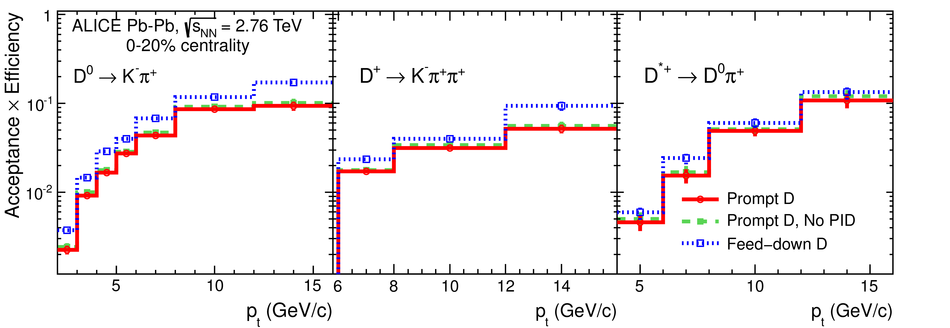## Figure 4

 Relative variation of the prompt $\Dzero$ meson yield as a function of the hypothesis on $\RAA^{\rm feed-down}/\RAA^{\rm prompt}$ for the B feed-down subtraction approach based on Eq. (3).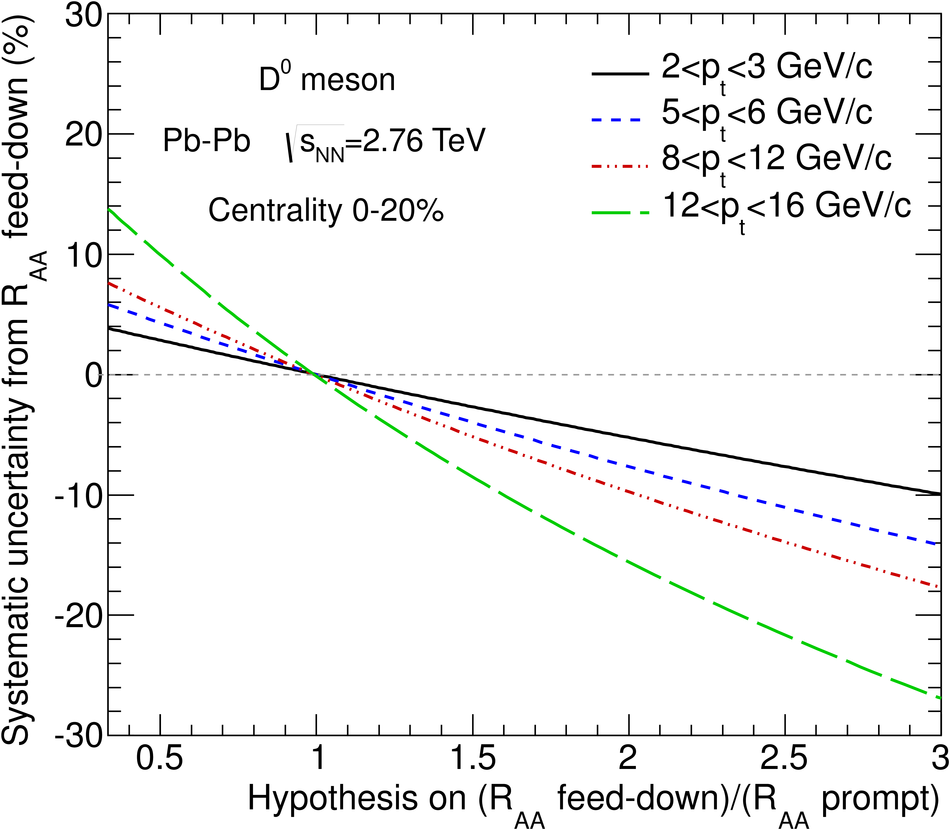## Figure 5

 Transverse momentum distributions d$N$/d$\pt$ of prompt $\rm D^0$ (left) and $\rm D^+$ (centre), and $\rm D^{*+}$ (right) mesons in the 0-20% and 40-80% centrality classes in Pb-Pb collisions at $\sqrtsNN=2.76~\tev$. The reference pp distributions $\langle{T_{\rm AA}}\rangle$d$\sigma$/d$\pt$ are shown as well. Statistical uncertainties (bars) and systematic uncertainties from data analysis (empty boxes) and from feed-down subtraction (full boxes) are shown. For Pb-Pb, the latter includes the uncertainties from the FONLL feed-down correction and from the variation of the hypothesis on$R_{{\rm AA}}^{\rm prompt} / R_{{\rm AA}}^{\rm feed-down}$. Horizontal error bars reflect bin widths, symbols were placed at the centre of the bin.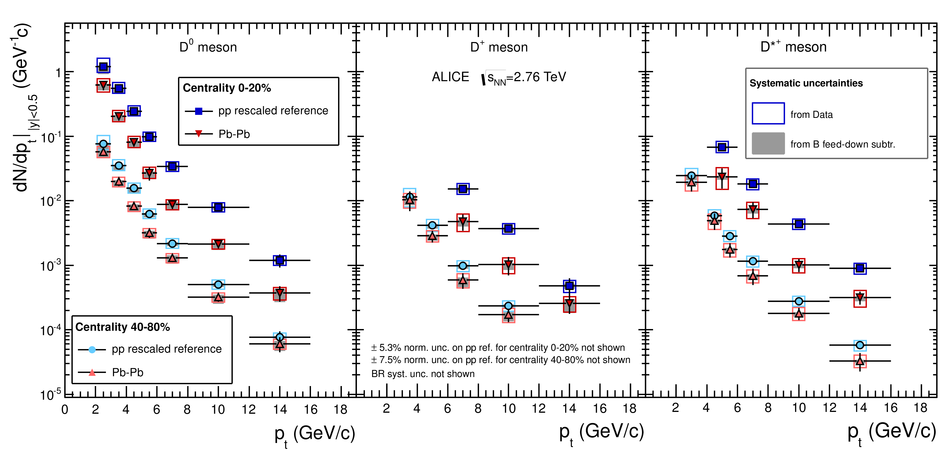## Figure 6

 $\RAA$ for prompt $\rm D^0$, $\rm D^+$, and $\Dstar$ in the 0--20\% (left) and 40-80% (right) centrality classes. Statistical (bars), systematic (empty boxes), and normalization (full box) uncertainties are shown. Horizontal error bars reflect bin widths, symbols were placed at the centre of the bin.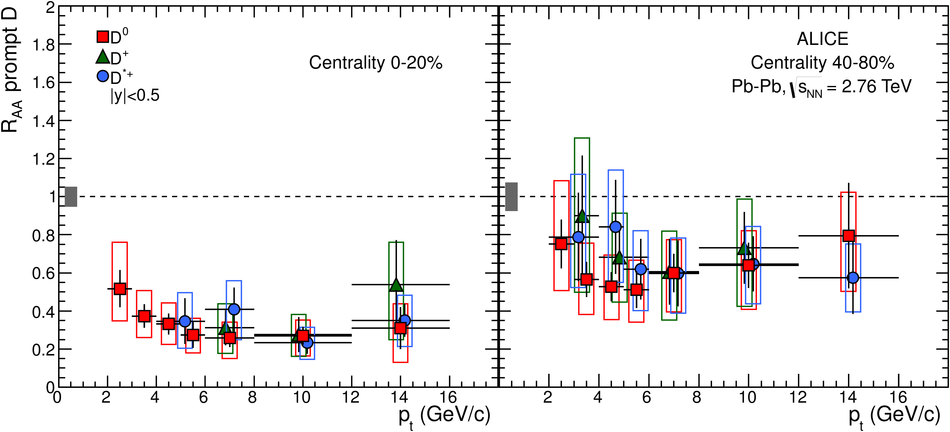## Figure 7

 Centrality dependence of $\RAA$ for prompt D mesons. Left: $\Dzero$ mesons with $2< \pt< 5~\gev/c$. Right: $\Dzero$, $\Dplus$, and $\Dstar$ mesons with $6< \pt< 12~\gev/c$ $\Dplus$ and $\Dstar$ points are displaced horizontally for better visibility.## Figure 8

 Average $\RAA$ of D mesons in the 0-20% centrality class compared to: left, the expectation from NLO pQCD  with nuclear shadowing ; right, the nuclear modification factors of charged particles  and non-prompt $\rm J/\psi$ from B decays  in the same centrality class. The charged particle $\RAA$ is shown only for $2< \pt< 16~\gev/c$. The three normalization uncertainties shownin the right-hand panel are almost fully correlated.## Figure 9

 Average $\RAA$ of D mesons (left) and $\RAA$ of charged particles (right) in the 0-20% centrality class compared to model calculations: (I) [13, 50], (II) , (III) , (IV) , (V) , (VI) , (VII) , (VIII) . The two normalization uncertainties are almost fully correlated.